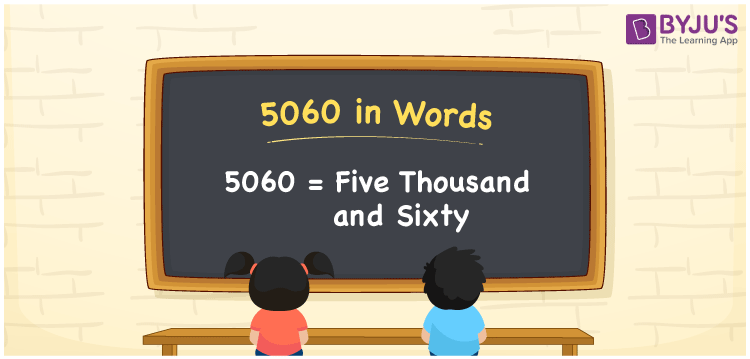# 5060 in Words

5060 in words can be written as Five Thousand and Sixty. Students will be able to learn the conversion of 5060 in words which will help them understand the applications of numbers in our daily lives. If you buy a watch for Rs. 5060, then you can say that “I have bought a watch for Five Thousand and Sixty Rupees”. The  number 5060 can be written in words using the English alphabet. The numbers in words can be grasped easily by the students using the resources given at BYJU’S. 5060 in English can be read as “Five Thousand and Sixty”.

 5060 in words Five Thousand and Sixty Five Thousand and Sixty in Numbers 5060

## 5060 in English Words## How to Write 5060 in Words?

Students will learn about the conversion of 5060 into words from place value charts. The number 5060 has four digits. For 5060, the place value chart is prepared in a table form to help students understand it effectively.

 Thousands Hundreds Tens Ones 5 0 6 0

5060 in expanded form is explained in brief here:

5 × Thousand + 0 × Hundred + 6 × Ten + 0 × One

= 5 × 1000  + 0 × 100 + 6 × 10 + 0 × 1

= 5000 + 000 + 60 + 0

= 5060

= Five Thousand and Sixty

Therefore, 5060 in words is written as Five Thousand and Sixty.

5060 is a natural number that precedes 5061 and succeeds 5059.

5060 in words – Five Thousand and Sixty

Is 5060 an odd number? – No

Is 5060 an even number? – Yes

Is 5060 a perfect square number? – No

Is 5060 a perfect cube number? – No

Is 5060 a prime number? – No

Is 5060 a composite number? – Yes

## Frequently Asked Questions on 5060 in Words

Q1

### How do you write 5060 in words?

5060 can be written as “Five Thousand and Sixty” in words.
Q2

### Is 5060 an even number?

5060 is an even number because it is divisible by 2.
Q3

### How can Five Thousand and Sixty be written in numbers?

Five Thousand and Sixty can be written in numbers as 5060.